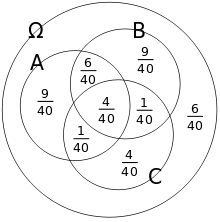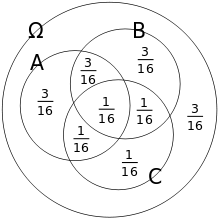# Independence (probability theory)

In probability theory, two events are independent, statistically independent, or stochastically independent if the occurrence of one does not affect the probability of the other. Similarly, two random variables are independent if the realization of one does not affect the probability distribution of the other.

The concept of independence extends to dealing with collections of more than two events or random variables, in which case the events are pairwise independent if each pair are independent of each other, and the events are mutually independent if each event is independent of each other combination of events.

## Definition

### For events

#### Two events

Two events A and B are independent (often written asor) if their joint probability equals the product of their probabilities:.

Why this defines independence is made clear by rewriting with conditional probabilities:.

and similarly.

Thus, the occurrence of B does not affect the probability of A, and vice versa. Although the derived expressions may seem more intuitive, they are not the preferred definition, as the conditional probabilities may be undefined if P(A) or P(B) are 0. Furthermore, the preferred definition makes clear by symmetry that when A is independent of B, B is also independent of A.

#### More than two events

A finite set of events {Ai} is pairwise independent if and only if every pair of events is independentthat is, if and only if for all distinct pairs of indices m, k,A finite set of events is mutually independent if and only if every event is independent of any intersection of the other eventsthat is, if and only if for every n-element subset {Ai},This is called the multiplication rule for independent events. Note that it is not a single condition involving only the product of all the probabilities of all single events (see below for a counterexample); it must hold true for all subset of events.

For more than two events, a mutually independent set of events is (by definition) pairwise independent; but the converse is not necessarily true (see below for a counterexample).

### For random variables

#### Two random variables

Two random variables X and Y are independent if and only if (iff) the elements of the π-system generated by them are independent; that is to say, for every a and b, the events {Xa} and {Yb} are independent events (as defined above). That is, X and Y with cumulative distribution functionsand, and probability densitiesand, are independent iff the combined random variable (X, Y) has a joint cumulative distribution functionor equivalently, if the joint density exists,#### More than two random variables

A set of random variables is pairwise independent if and only if every pair of random variables is independent.

A set of random variables is mutually independent if and only if for any finite subsetand any finite sequence of numbers, the eventsare mutually independent events (as defined above).

The measure-theoretically inclined may prefer to substitute events {XA} for events {Xa} in the above definition, where A is any Borel set. That definition is exactly equivalent to the one above when the values of the random variables are real numbers. It has the advantage of working also for complex-valued random variables or for random variables taking values in any measurable space (which includes topological spaces endowed by appropriate σ-algebras).

#### Conditional independence

Intuitively, two random variables X and Y are conditionally independent given Z if, once Z is known, the value of Y does not add any additional information about X. For instance, two measurements X and Y of the same underlying quantity Z are not independent, but they are conditionally independent given Z (unless the errors in the two measurements are somehow connected).

The formal definition of conditional independence is based on the idea of conditional distributions. If X, Y, and Z are discrete random variables, then we define X and Y to be conditionally independent given Z iffor all x, y and z such that P(Z = z) > 0. On the other hand, if the random variables are continuous and have a joint probability density function p, then X and Y are conditionally independent given Z iffor all real numbers x, y and z such that pZ(z) > 0.

If X and Y are conditionally independent given Z, thenfor any x, y and z with P(Z = z) > 0. That is, the conditional distribution for X given Y and Z is the same as that given Z alone. A similar equation holds for the conditional probability density functions in the continuous case.

Independence can be seen as a special kind of conditional independence, since probability can be seen as a kind of conditional probability given no events.

### Independent σ-algebras

The definitions above are both generalized by the following definition of independence for σ-algebras. Let (Ω, Σ, Pr) be a probability space and let A and B be two sub-σ-algebras of Σ. A and B are said to be independent if, whenever A  A and B  B,Likewise, a finite family of σ-algebrasis said to be independent if and only ifand an infinite family of σ-algebras is said to be independent if all its finite subfamilies are independent.

The new definition relates to the previous ones very directly:

• Two events are independent (in the old sense) if and only if the σ-algebras that they generate are independent (in the new sense). The σ-algebra generated by an event E  Σ is, by definition,• Two random variables X and Y defined over Ω are independent (in the old sense) if and only if the σ-algebras that they generate are independent (in the new sense). The σ-algebra generated by a random variable X taking values in some measurable space S consists, by definition, of all subsets of Ω of the form X−1(U), where U is any measurable subset of S.

Using this definition, it is easy to show that if X and Y are random variables and Y is constant, then X and Y are independent, since the σ-algebra generated by a constant random variable is the trivial σ-algebra {∅, Ω}. Probability zero events cannot affect independence so independence also holds if Y is only Pr-almost surely constant.

## Properties

### Self-independence

Note that an event is independent of itself if and only if.

Thus an event is independent of itself if and only if it almost surely occurs or its complement almost surely occurs.

### Expectation and covariance

If X and Y are independent, then the expectation operator E has the propertyand the covariance cov(X, Y) is zero, since we have(The converse of these, i.e. the proposition that if two random variables have a covariance of 0 they must be independent, is not true. See uncorrelated.)

### Characteristic function

Two random variables X and Y are independent if and only if the characteristic function of the random vector (X, Y) satisfiesIn particular the characteristic function of their sum is the product of their marginal characteristic functions:though the reverse implication is not true. Random variables that satisfy the latter condition are called subindependent.

## Examples

### Rolling dice

The event of getting a 6 the first time a die is rolled and the event of getting a 6 the second time are independent. By contrast, the event of getting a 6 the first time a die is rolled and the event that the sum of the numbers seen on the first and second trials is 8 are not independent.

### Drawing cards

If two cards are drawn with replacement from a deck of cards, the event of drawing a red card on the first trial and that of drawing a red card on the second trial are independent. By contrast, if two cards are drawn without replacement from a deck of cards, the event of drawing a red card on the first trial and that of drawing a red card on the second trial are again not independent.

### Pairwise and mutual independencePairwise independent, but not mutually independent, events.Mutually independent events.

Consider the two probability spaces shown. In both cases, P(A) = P(B) = 1/2 and P(C) = 1/4 The first space is pairwise independent but not mutually independent. The second space is both pairwise independent and mutually independent. To illustrate the difference, consider conditioning on two events. In the pairwise independent case, although any one event is independent of each of the other two individually, it is not independent of the intersection of the other two:In the mutually independent case however:### Mutual independence

It is possible to create a three-event example in whichand yet no two of the three events are pairwise independent (and hence the set of events are not mutually independent). This example shows that mutual independence involves requirements on the products of probabilities of all combinations of events, not just the single events as in this example.